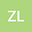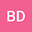The Schwarz lemma in bicomplex analysis
••Binlin Dai
Shanghai University of Finance and Economics
Author Profile## Abstract

We investigate Schwarz lemma in the framework of bicomplex numbers, which are pairs of complex numbers making up a commutative ring with zero-divisors. The bicomplex is a generalization of complex which has closed relation with Frac- tal geometry, Minkowski Space-Time, Maxwell’s equations, Schrödinger equation and Gaussian pulse wave. In this paper, we first construct a type of bicomplex Möbius transformation and obtain some results : the mapping properties on bicom- plex spheres and bicomplex ball, preserving the inverse points with respect to the bicomplex sphere (0,1). Then we obtain the Poisson integral formula in bicom- plex setting, and by using the Poisson integral formula, we give the Schwarz lemma for bicomplex holomorphic functions in bicomplex setting. Finally, we shall give the Schwarz lemma and the Schwarz-Pick type lemma for holomorphic functions in bicomplex analysis. These results may give new energy for the development of quan- tum mechanics.
20 Apr 2022Submitted to Mathematical Methods in the Applied Sciences
20 Apr 2022Submission Checks Completed
20 Apr 2022Assigned to Editor
03 May 2022Reviewer(s) Assigned
09 Aug 2022Review(s) Completed, Editorial Evaluation Pending
15 Aug 2022Editorial Decision: Revise Major